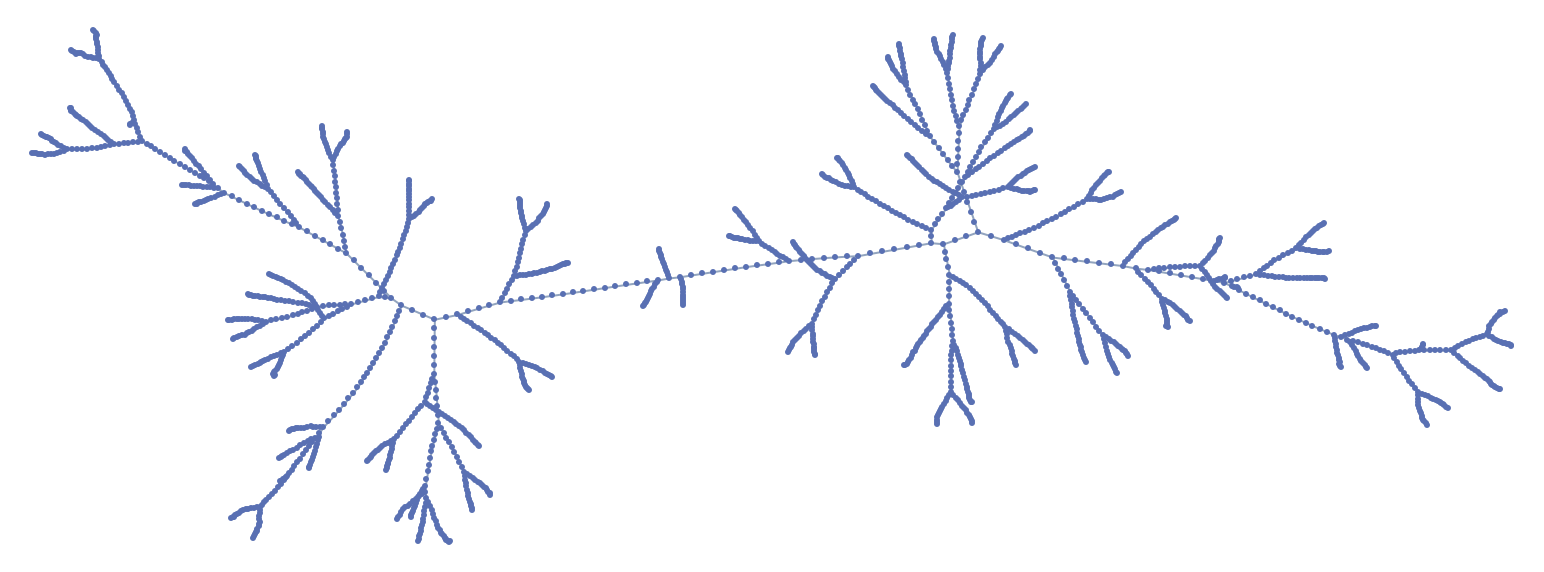#Function Repository Resource:

# DiskPoints

Get uniformly distributed points on a disk

Contributed by: Sander Huisman  |  SHuisman
 ResourceFunction["DiskPoints"][n] gives the positions of n uniformly distributed points on a unit disk.

## Details and Options

The points are distributed using Vogel's phyllotaxis method.

## Examples

### Basic Examples (2)

500 uniformly distributed points on a unit disk:

 In:=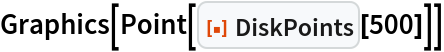Out=Compare this with the boundaries of a unit disk:

 In:=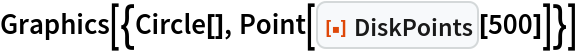Out=### Applications (1)

Use DiskPoints to make a uniform mesh:

 In:=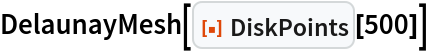Out=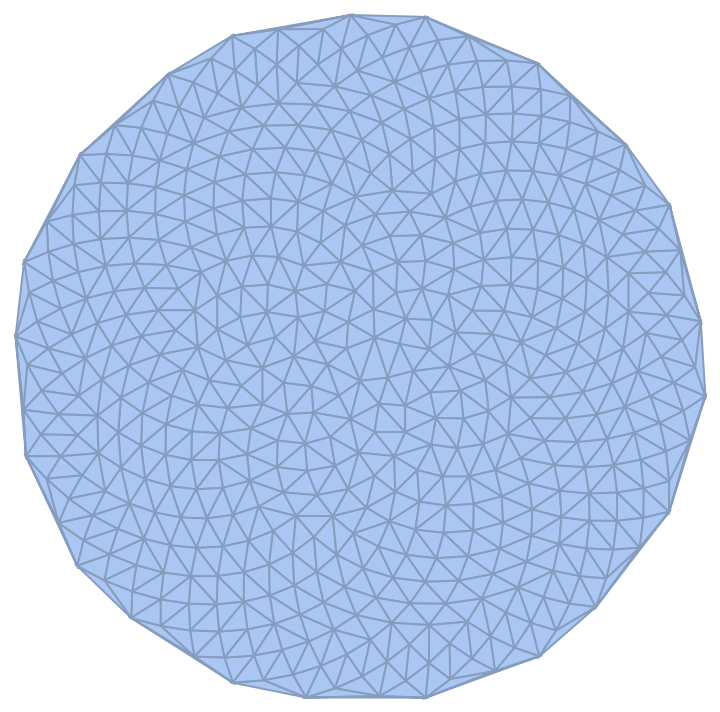### Neat Examples (3)

Connect each point to its nearest neighbor:

 In:=Out=Highlight the non-uniformity in the area determined using Voronoi analysis:

 In:=Out=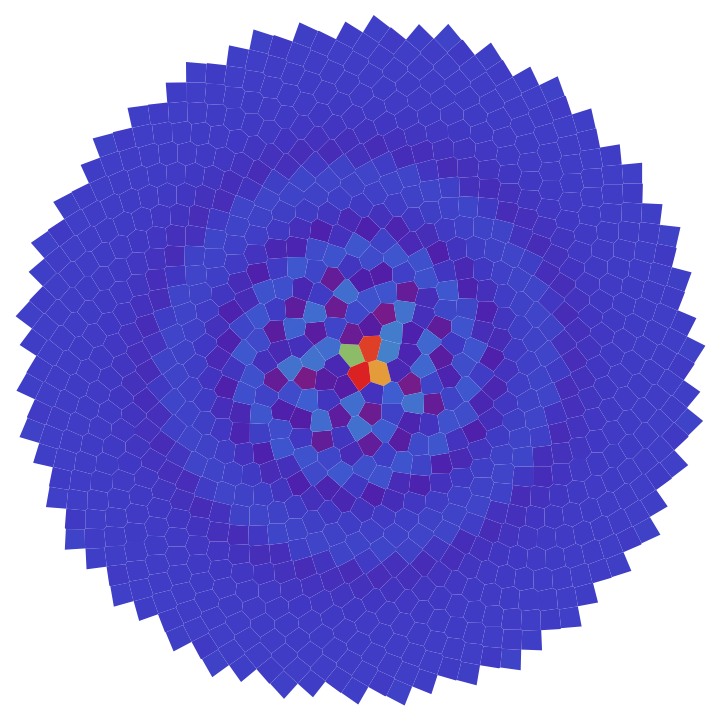Find the minimum spanning tree for 2000 points, and show the connectedness:

 In:=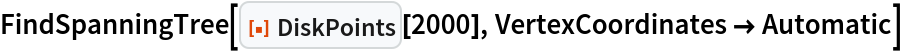Out=